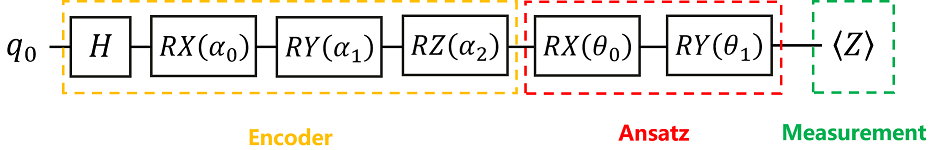# 量子神经网络初体验¶

## 量子神经网络的结构¶

（1）一个（或多个）编码线路，用于将经典数据编码到量子数据（通常称为Encoder）；

（2）一个（或多个）训练线路，用于训练带参量子门中的参数（通常称为Ansatz）；

（3）一个（或多个）测量，用于检测测量值（例如在Z方向上测量，就是某个量子比特的量子态在Z轴上的投影，该测量得到的是量子态关于泡利Z算符（不限定于泡利Z算符，换成其它的算符亦可）的期望值）是否接近于目标期望值。## 简单的例子¶$\langle 0|Z|0\rangle=1.$

## 环境准备¶

:

import numpy as np                                  # 导入numpy库并简写为np
from mindquantum.core.circuit import Circuit        # 导入Circuit模块，用于搭建量子线路
from mindquantum.core.gates import H, RX, RY, RZ    # 导入量子门H, RX, RY, RZ


## 搭建Encoder¶

:

# pylint: disable=W0104
encoder = Circuit()                   # 初始化量子线路
encoder += H.on(0)                    # H门作用在第0位量子比特
encoder += RX(f'alpha{0}').on(0)      # RX(alpha_0)门作用在第0位量子比特
encoder += RY(f'alpha{1}').on(0)      # RY(alpha_1)门作用在第0位量子比特
encoder += RZ(f'alpha{2}').on(0)      # RZ(alpha_2)门作用在第0位量子比特
encoder.as_encoder()                  # 将量子线路设置为encoder线路
encoder.summary()                     # 总结Encoder
encoder.svg()

==================Circuit Summary==================
|Total number of gates  : 4.                      |
|Parameter gates        : 3.                      |
|with 3 parameters are  : alpha0, alpha1, alpha2. |
|Number qubit of circuit: 1                       |
===================================================

::

alpha0, alpha1, alpha2 = 0.2, 0.3, 0.4              # alpha0, alpha1, alpha2为已知的固定值，分别赋值0.2, 0.3 和0.4
state = encoder.get_qs(pr={'alpha0': alpha0, 'alpha1': alpha1, 'alpha2': alpha2}, ket=True)
print(state)

(0.5669903122552596-0.1753906567580312j)¦0⟩
(0.800814626197614+0.08034947292077024j)¦1⟩


（1）通过调用量子线路的get_qs函数，我们能够得到该量子线路在全零态基础上演化出来的量子态。

（2）get_qspr参数代表变分量子线路中的参数值，ket表示是否将量子态输出为右矢形式。

## 搭建Ansatz¶

:

# pylint: disable=W0104
ansatz = Circuit()                           # 初始化量子线路
ansatz += RX(f'theta{0}').on(0)              # RX(theta_0)门作用在第0位量子比特
ansatz += RY(f'theta{1}').on(0)              # RY(theta_1)门作用在第0位量子比特
ansatz.as_ansatz()                           # 将量子线路设置成待训练线路
ansatz.svg()                                 # 打印量子线路

::

theta0, theta1 = 0, 0                        # 对theta0, theta1进行赋值，设为初始值0, 0
state = ansatz.get_qs(pr=dict(zip(ansatz.params_name, [theta0, theta1])), ket=True)
print(state)

1¦0⟩


:

# pylint: disable=W0104
circuit = encoder.as_encoder() + ansatz.as_ansatz()                   # 完整的量子线路由Encoder和Ansatz组成
circuit.svg()

:## 构建哈密顿量¶

:

from mindquantum.core.operators import QubitOperator           # 导入QubitOperator模块，用于构造泡利算符
from mindquantum.core.operators import Hamiltonian             # 导入Hamiltonian模块，用于构建哈密顿量

ham = Hamiltonian(QubitOperator('Z0', -1))                     # 对第0位量子比特执行泡利Z算符测量，且将系数设置为-1，构建对应的哈密顿量
print(ham)

-1 [Z0]


$\langle 1|Z|1\rangle=-1.$

$\langle 0|(-Z)|0\rangle=-1.$

（1）QubitOperator是作用于量子比特的算子的总和，主要用于构造泡利算符；一般格式如下：QubitOperator(term=None, coefficient=1.0)；

（2）Hamiltonian是哈密顿量包装器，主要用于构建哈密顿量，一般格式如下：Hamiltonian(QubitOperator(‘X0 Y2’, 0.5))，X0和Y2表示泡利X算符作用在第0位量子比特，泡利Y算符作用在第2位量子比特，系数为0.5。

## 生成变分量子线路模拟算子¶

:

encoder_names = encoder.params_name                   # Encoder中所有参数组成的数组，encoder.para_name系统会自动生成
ansatz_names = ansatz.params_name                     # Ansatz中所有参数组成的数组，ansatz.para_name系统会自动生成

print('encoder_names = ', encoder.params_name, '\nansatz_names =', ansatz.params_name)

encoder_names =  ['alpha0', 'alpha1', 'alpha2']
ansatz_names = ['theta0', 'theta1']


:

# 导入Simulator模块
from mindquantum.simulator import Simulator

# 生成一个基于mqvector后端的模拟器，并设置模拟器的比特数为量子线路的比特数。
sim = Simulator('mqvector', circuit.n_qubits)

# 获取模拟器基于当前量子态的量子线路演化以及期望、梯度求解算子
circuit)

# Encoder中的alpha0, alpha1, alpha2这三个参数组成的数组，
# 将其数据类型转换为float32，并储存在encoder_data中。
# MindSpore Quantum支持多样本的batch训练，Encoder数组是两个维度，
# 第一个维度为样本，第二个维度为特征（即参数）
encoder_data = np.array([[alpha0, alpha1, alpha2]]).astype(np.float32)

# Ansatz中的theta0, theta1这两个参数组成的数组，将其数据类型转换为float32，
# 并储存在ansatzr_data中，Ansatz数据只有一个维度，特征（即参数）
ansatz_data = np.array([theta0, theta1]).astype(np.float32)

# 根据Encoder和Ansatz的数据，输出变分量子线路的测量值，Encoder中的参数的导数和Ansatz中的参数的导数

print('Measurement result: ', measure_result)

Measurement result:  [[0.29552022+0.j]]
Gradient of encoder parameters:  [[[0.+0.j 0.+0.j 0.+0.j]]]
Gradient of ansatz parameters:  [[[-0.37202556+0.j  0.87992317+0.j]]]


（1）Simulatorget_expectation_with_grad用于生成变分量子线路来模拟算子，一般格式如下：

Simulator.get_expectation_with_grad(ham,
circ_right,
circ_left,
parallel_worker=1)


$E=\left<0\right|U^\dagger_l(\theta) H U_r(\theta)\left|0\right>$

（2）MindSpore是一个全场景深度学习框架，旨在实现易开发、高效执行、全场景覆盖三大目标，提供支持异构加速的张量可微编程能力，支持云、服务器、边和端多种硬件平台。

## 搭建量子神经网络¶

:

# pylint: disable=W0104
from mindquantum.framework import MQLayer          # 导入MQLayer
import mindspore as ms                             # 导入mindspore

ms.set_seed(1)                                     # 设置生成随机数的种子
ms.set_context(mode=ms.PYNATIVE_MODE, device_target="CPU")

QuantumNet

:

MQLayer<
(evolution): MQOps<1 qubit mqvector VQA Operator>
>


（1）MindSpore Quantum中的量子线路梯度计算算子都是在PYNATIVE_MODE下的，因此需要设置MindSpore的运行模式。

（2）我们也可以通过如下代码方式搭建量子机器学习层，只是在MindSpore Quantum中，已经将下述过程封装打包，这样我们就可以直接利用MQLayer模块搭建量子机器学习层。对于更复杂的量子-经典混合神经网络，如下搭建方式会展示它的优势。

from mindspore import nn

class MQLayer(nn.Cell):
super(MQLayer, self).__init__()
weight_size = len(
self.weight = Parameter(initializer(weight,
weight_size,
dtype=ms.float32),
name='ansatz_weight')

def construct(self, x):
return self.evolution(x, self.weight)


## 训练¶

:

from mindspore.nn import Adam, TrainOneStepCell                   # 导入Adam模块和TrainOneStepCell模块

opti = Adam(QuantumNet.trainable_params(), learning_rate=0.5)     # 需要优化的是Quantumnet中可训练的参数，学习率设为0.5
net = TrainOneStepCell(QuantumNet, opti)

for i in range(200):
res = net(ms.Tensor(encoder_data))
if i % 10 == 0:
print(i, ': ', res)

0 :  [[0.2837115]]
10 :  [[-0.8851233]]
20 :  [[-0.97001773]]
30 :  [[-0.9929431]]
40 :  [[-0.9939507]]
50 :  [[-0.9967015]]
60 :  [[-0.99878186]]
70 :  [[-0.9995535]]
80 :  [[-0.9999011]]
90 :  [[-0.99998033]]
100 :  [[-0.9999989]]
110 :  [[-0.99999785]]
120 :  [[-0.999997]]
130 :  [[-0.9999987]]
140 :  [[-0.9999998]]
150 :  [[-1.]]
160 :  [[-0.99999994]]
170 :  [[-1.]]
180 :  [[-1.]]
190 :  [[-1.]]


（2）TrainOneStepCell模块为网络训练包类，用优化器包装网络。生成的单元格使用输入“inputs”进行训练，将在构造函数中创建反向图，以更新参数，有不同的并行模式可用于训练。一般格式如下：nn.TrainOneStepCell(network, optimizer, sens=1.0)。

## 结果呈现¶

:

theta0, theta1 = QuantumNet.weight.asnumpy()

print(QuantumNet.weight.asnumpy())

[ 2.2420275 -1.0756909]


:

pr = {'alpha0': alpha0, 'alpha1': alpha1, 'alpha2': alpha2, 'theta0': theta0, 'theta1': theta1}
state = circuit.get_qs(pr=pr, ket=True)

print(state)

(0.37129760050057437-0.9285139157007681j)¦0⟩
(1.4564552975271372e-05+6.455516706194153e-07j)¦1⟩


:

state = circuit.get_qs(pr=pr)
fid = np.abs(np.vdot(state, [1, 0]))**2            # 保真度fidelity为向量内积的绝对值的模平方，即计算此时量子态对应的向量与|0>态对应的向量[1,0]的内积的模平方

print(fid)

0.9999999997874573